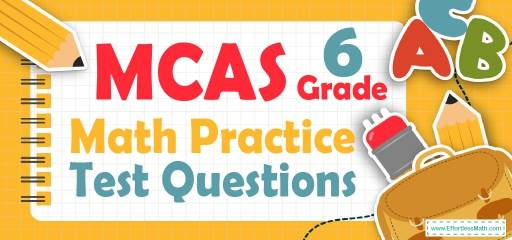# 6th Grade MCAS Math Practice Test QuestionsIt may seem difficult to get a great score on the 6th Grade MCAS Math exam, but we will make it easy for your students! Your 6th Grade students can greatly improve their math skills by solving the 10 most common 6th Grade MCAS Math test questions in this article. The exact solution of the questions is also given at the end of the questions to help them prevent further mistakes.

Make sure to follow some of the related links at the bottom of this post to get a better idea of what kind of mathematics questions students need to practice.

The Absolute Best Book to Ace the MCAS Math Test

## 10 Sample 6th Grade MCAS Math Practice Questions

1- What is the missing prime factor of number 420?
$$420=2^2×3^1×…$$

A. $$2^2×3^1×5^1×7^1$$

B. $$2^2×3^1×7^1×9^1$$

C. $$1^2×2^3×2^1×3^1$$

D. $$3^2×5^1×7^1×9^1$$

2- If the area of the following trapezoid is equal to $$A$$, which equation represents $$x$$?

A. $$x = \frac{13}{A}$$

B. $$x = \frac{A}{13}$$

C. $$x=A+13$$

D. $$x=A-13$$

3- By what factor did the number below change from first to the fourth number?
$$8, 104, 1352, 17576$$

A. 13

B. 96

C. 1456

D. 17568

4- 170 is equal to …

A. $$-20-(3×10)+(6×40)$$

B. $$((\frac{15}{8})×72 )+ (\frac{125}{5})$$

C. $$((\frac{30}{4} + \frac{15}{2})×8) – \frac{11}{2} + \frac{222}{4}$$

D. $$\frac{481}{6} + \frac{121}{3}+50$$

5- The distance between the two cities is 3,768 feet. What is the distance between the two cities in yards?

A. 1,256 yd

B. 11,304 yd

C. 45,216 yd

D. 3,768 yd

6- Mr. Jones saves $3,400 out of his monthly family income of$74,800. What fractional part of his income does Mr. Jones save?

A. $$\frac{1}{22}$$

B. $$\frac{1}{11}$$

C. $$\frac{3}{25}$$

D. $$\frac{2}{15}$$

7- What is the lowest common multiple of 12 and 20?

A. 60

B. 40

C. 20

D. 12

8- Based on the table below, which expression represents any value of f in term of its corresponding value of $$x$$?

A. $$f=2x-\frac{3}{10}$$

B. $$f=x+\frac{3}{10}$$

C. $$f=2x+2 \frac{2}{5}$$

D. $$2x+\frac{3}{10}$$

9- 96 kg $$=$$… ?

A. 96 mg

B. 9,600 mg

C. 960,000 mg

D. 96,000,000 mg

10- Calculate the approximate area of the following circle? (the diameter is 25)

A. 78

B. 491

C. 157

D. 1963

## Best 6th Grade MCAS Math Prep Resource for 2022

1- A
$$420=2^2×3^1×5^1×7^1$$

2- B
The area of the trapezoid is: area= $$\frac{(base 1+base 2)}{2})×height= ((\frac{10 + 16}{2})x = A$$
$$→13x = A→x = \frac{A}{13}$$

3- A
$$\frac{104}{8}=13, \frac{1352}{104}=13, \frac{17576}{1352}=13$$
Therefore, the factor is 13

4- C
Simplify each option provided.
$$A. -20-(3×10)+(6×40)=-20-30+240=190$$
$$B. (\frac{15}{8})×72 + (\frac{125}{5}) =135+25=160$$
$$C. ((\frac{30}{4} + \frac{15}{2})×8) – \frac{11}{2} + \frac{222}{4} = ((\frac{30 + 30}{4})×8)- \frac{11}{2}+ \frac{111}{2}=(\frac{60}{4})×8) + \frac{100}{2}= 120 + 50 = 170$$this is the answer
$$D. \frac{481}{6} + \frac{121}{3}+50= \frac{481+242}{6}+50=120.5+50=170.5$$

5- A
1 yard $$=$$3 feet
Therefore, $$3,768 ft × \frac{1 \space yd }{3 \space ft}=1,256 \space yd$$

6- A
3,400 out of 74,800 equals to $$\frac{3,400}{74,800}=\frac{17}{374}=\frac{1}{22}$$

7- A
Prime factorizing of $$20=2×2×5$$
Prime factorizing of $$12=2×2×3$$
LCM$$=2×2×3×5=60$$

8- C
Plug in the value of $$x$$ into the function f. First, plug in 3.1 for $$x$$.
$$A. f=2x-\frac{3}{10}=2(3.1)-\frac{3}{10}=5.9≠8.6$$
$$B. f=x+\frac{3}{10}=3.1+\frac{3}{10}=3.4≠10.8$$
$$C. f=2x+2 \frac{2}{5}=2(3.1)+2 \frac{2}{5}=6.2+2.4=8.6$$
This is correct!
Plug in other values of $$x. x=4.2$$
$$f=2x+2\frac{2}{5} =2(4.2)+2.4=10.8$$
This one is also correct.
$$x=5.9$$
$$f=2x+2 \frac{2}{5}=2(5.9)+2.4=14.2$$
This one works too!
$$D. 2x+\frac{3}{10}=2(3.1)+\frac{3}{10}=6.5≠8.6$$

9- D
1 kg$$=$$ 1000 g and 1 g $$=$$ 1000 mg
96 kg$$=$$ 96 $$×$$ 1000 g $$=$$96 $$×$$ 1000 $$×$$ 1000 $$=$$96,000,000 mg

10- B
The diameter of a circle is twice the radius. Radius of the circle is $$\frac{25}{2}$$.
Area of a circle = $$πr^2=π(\frac{25}{2})^2=156.25π=156.25×3.14=490.625≅491$$

Looking for the best resource to help you succeed on the MCAS Math Grade 6 Math test?

The Most Comprehensive Review for 6th-Grade Students

### What people say about "6th Grade MCAS Math Practice Test Questions - Effortless Math: We Help Students Learn to LOVE Mathematics"?

No one replied yet.

X
51% OFF

Limited time only!

Save Over 51%

SAVE $15 It was$29.99 now it is \$14.99